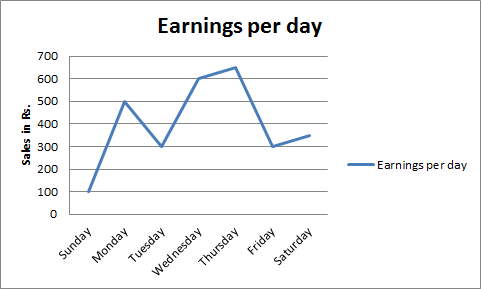# Data Interpretation - Line Charts

>>>>>>>>Line Charts

Instructions:
Directions. The graph given below represents the variations in earnings in rupees over a week. Study the graph and answer questions given below• A

Sunday-Monday• B

Tuesday-Wednesday• C

Thursday-Friday• D

None of theseInstructions:
Directions. The graph given below represents the variations in earnings in rupees over a week. Study the graph and answer questions given below

• A

Monday• B

Wednesday• C

Thursday• D

SaturdayInstructions:
Directions. The graph given below represents the variations in earnings in rupees over a week. Study the graph and answer questions given below

• A

Rs. 325/-• B

Rs. 400/-• C

Rs. 270/-• D

Rs. 375/-Instructions:
Directions. The graph given below represents the variations in earnings in rupees over a week. Study the graph and answer questions given below

• A

Unequal• B

Equal• C

More• D

LessInstructions:
Directions. The graph given below represents the variations in earnings in rupees over a week. Study the graph and answer questions given below

• A

Saturday• B

Sunday• C

Wednesday-Thursday• D

None of theseRelated Quiz.
Line Charts